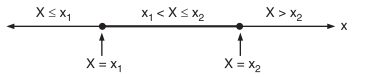# Cumulative Distribution Function (CDF)

## Cumulative Distribution Function (CDF)

The cumulative distribution function (CDF) of a random variable is defined as the probability that the random variable X takes value less than or equal to x.

i.e.,        CDF FX(x) = P(X ≤ x) …………….(1)

Here, x is a dummy variable and the CDF is denoted by FX(x).

It is possible to define CDF for continuous as well as the discrete random variables. The CDF is sometimes called as simply the distribution function.

### Important Properties of CDF

Property 1:  The CDF is always bounded between 0 and 1.

i.e.,          0 ≤ FX(x) ≥ 1 ………….(2)

As per the definition of CDF, it is a probability function P(X ≤ x) and any probability must have a value between 0 and 1. Therefore, CDF is always bounded between 0 and 1.

Property 2: This property states that,

FX(∞)= 1 …………(3)

Proof: Here, FX(∞) = P (X ≤ ∞). This includes the probability of all the possible outcomes or events. The random variable X ≤ ∞, thus, becomes a ‘certain event’ and therefore has a 100% probability.

Property 3: This property states that,

FX(-∞) = 0 ……………..(6.18)

Proof: Here, FX(-∞) = P(X ≤ -∞). The random variable X cannot have any value which is less than or equal to – ∞. Thus, X ≤ -∞ is a null event and therefore, has a 0% probability.

Property 4: This property states that FX(x) is a monotone non-decreasing function i.e.,

FX(x1) ≤ FX(x2)    for x1 < x2 ……………….(4)

Proof: To prove this property, let us consider fig.1.Fig.1

FX(x2) is defined as under: FX(x2) = P(X ≤ x2)

The R.H.S of this equation can be expressed as union of two probabilities.

Therefore,

FX(x2) = P(X ≤ x2) = P(X ≤ x1) ∪ P (x1 < X ≤ x2) ………………. (5)

The two events X ≤ x1 and x1 < X ≤ x2 are mutually exclusive.

Therefore, we write

P(X ≤ x1) ∪ P (x1 < X ≤ x2) = P(X ≤ x1) + P (x1 < X ≤ x2)

Substituting this value in equation (5), we get

FX(x2) = P(X ≤ x1) + P(x1 < X ≤ x2) …………….(6)

But, the first term in equation (6) i.e., P(X ≤ x1) = FX(x1)

Therefore,

FX(x2) = FX(x1) + P(x1 < X ≤ x2) ………………(7)

P(x1 < X ≤ x2) is always non-negative as it is a probability function, Thus,

FX(x2) ≥ FX(x1)

or FX(x1) ≤ FX(x2)     for x1 ≤ x2

These properties of CDF are general and are valid for the continuous as well as discrete random variables.